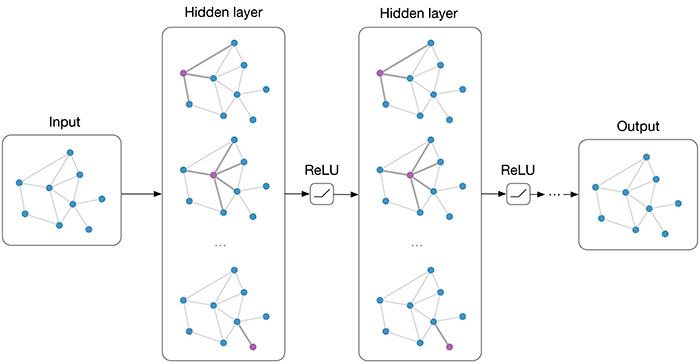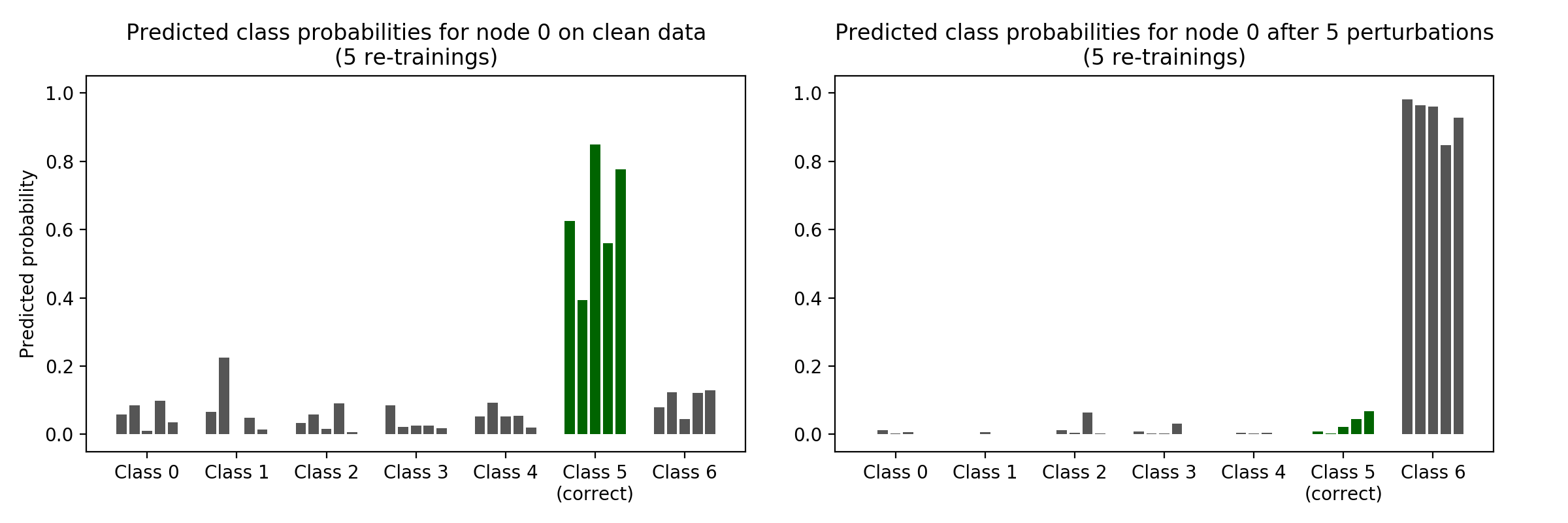# Graph Convolutional Network (GCN), Graph Neural Networks (Graph Nets), Geometric Deep Learning

A graph neural network is the "blending powerful deep learning approaches with structured representation" models of collections of objects, or entities, whose relationships are explicitly mapped out as "edges" connecting the objects. Human cognition makes the strong assumption that the world is composed of objects and relations, and because GNs [graph networks] make a similar assumption, their behavior tends to be more interpretable. Rather, modeling the relationships of objects is something that not only spans all the various machine learning models -- CNNs, recurrent neural networks (RNNs), long-short-term memory (LSTM) systems, etc. -- but also other approaches that are not neural nets, such as set theory. The idea is that graph networks are bigger than any one machine-learning approach. Graphs bring an ability to generalize about structure that the individual neural nets don't have. Graphs, generally, are a representation which supports arbitrary (pairwise) relational structure, and computations over graphs afford a strong relational inductive bias beyond that which convolutional and recurrent layers can provide. Google ponders the shortcomings of machine learning | Tiernan Ray## Graph Convolutional Network (GCN)## Graph Gated Neural Network (GGNN)## Adversarial Attacks on Neural Networks for Graph Data